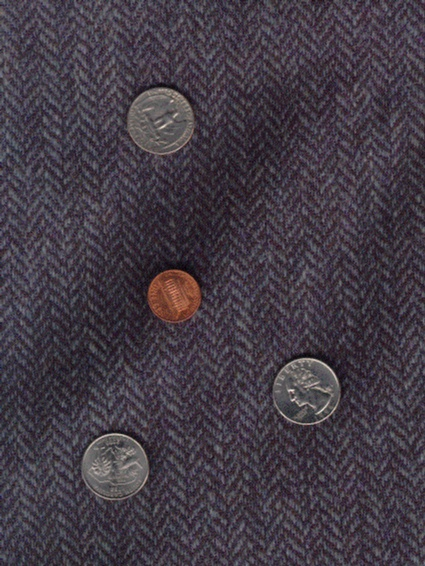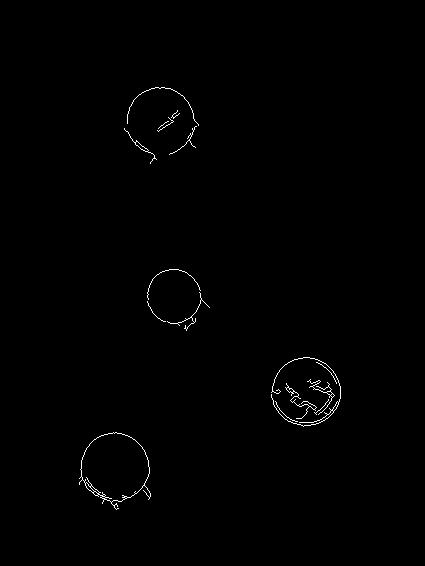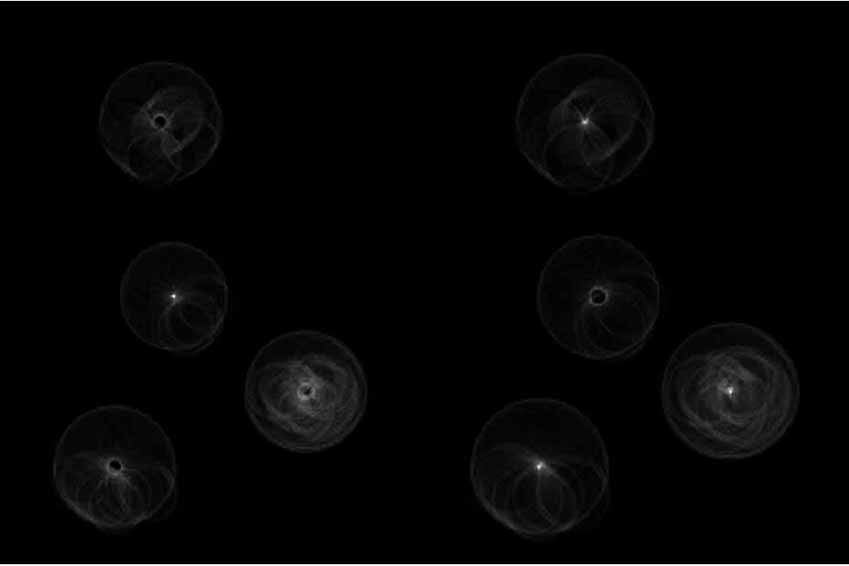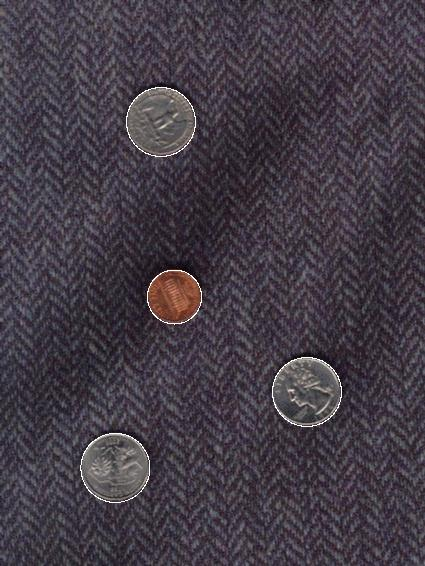Detecting Coins using Hough Tramsform

Vivek Kwatra

The problem was to detect coins in an image using edge detection and Hough Transform.
The technique was implemented in MATLAB. This page contains a description of the technique and images showing results.
I did the detection on the image coins2.jpg - the image with coins on a texture.

The function coin_detect is called with the filename of the image to detect the coins. It does the following -

1. Doing Edge detection on the image - I used the Canny edge detector with 0.1 and 0.45 as the low and high thresholds. This removed most of the noise due to the texture leaving only the edges of the coins and due to the texture within the coins.

3. Detecting circles - Once the hough transform image for a particular radius is computed, it is adjusted to lie between 0 and 1 and thresholded, so as to leave only those points with high probability of being the centers. The function mark_circles does this with a value of 0.67. The resulting point-sets are then labeled with different regions. The centroids of each region are considered as centers of the detected coins. The output image is computed by drawing circles with these points as centers and the matched radius as the radius, and adding this to the input image.

Discussion -  The technique works pretty well with this image. The computational complexity highly depends on the number of edge pixles and the number of radii to be matched. In this case the number of radii is pretty less. For the image considered, the edge pixels are also less because the texture does not introduce strong edges. For the second image it would be harder because the background introduces a lot of edges, increasing the number of edge pixels tremendously. Also it can falsely detect circles with similar radii if even if they are not coins.Original ImageEdge ImageHough Transform : Left for Penny, Right for QuartersDetected Coins